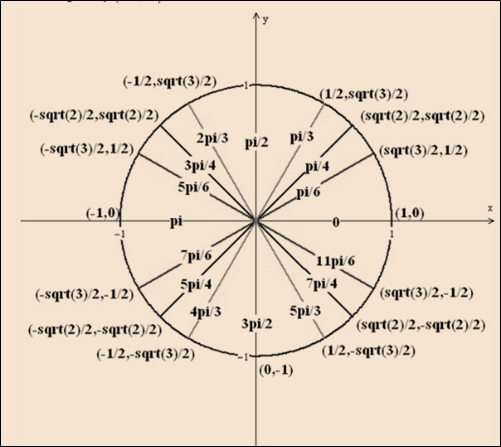# The value of x to satisfy the following inequality equation: sin x &gt; cos x### Single Variable Calculus: Concepts...

4th Edition
James Stewart
Publisher: Cengage Learning
ISBN: 9781337687805### Single Variable Calculus: Concepts...

4th Edition
James Stewart
Publisher: Cengage Learning
ISBN: 9781337687805

#### Solutions

Chapter C, Problem 36E
To determine

## To calculate: The value of x to satisfy the following inequality equation:   sinx>cosx

Expert Solution

The values of x for the interval [0,2π] to satisfy eq. sinx>cosx are [π4x5π4]

### Explanation of Solution

Given information:

sinx>cosx[0,2π]

Calculation:

Consider;

The unit circle with co-ordinates (x,y)=(cosx,sinx) and radius 1 to find out the values of x for the interval [0,2π] that cause sinx>cosx .Fig. Unit circle

From the figure;

sinx>cosx , in the second quadrant x is negative and y is positive. So, in second quadrant the given inequality equation is satisfied.

In the fourth quadrant sinx>cosx , no value of x satisfy the inequality equation because sine is negative and cosine is positive here.